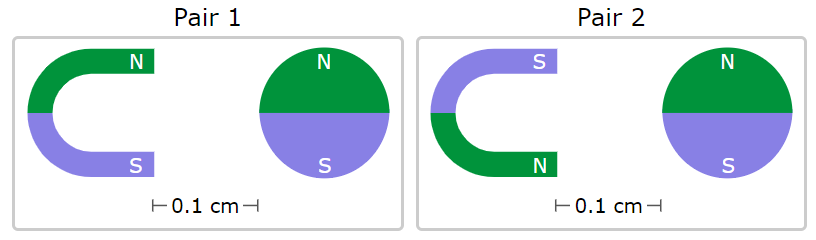# Understanding the Velocity, Acceleration, and Forces

In this quiz, you will get to know about the basic concept of velocities, accelerations, and forces. You will find questions on finding velocity, distance, and time. You will also learn about balanced and unbalanced forces.

Start Quiz

An earthworm crawled through a garden at a steady speed. It crawled 14 millimeters in 2 seconds. What was the earthworm's speed?

6

7

3

2

A cloud drifted across the sky at a steady speed. It moved 760 meters in 4 minutes. What was the cloud's speed?

190 meters per minute

160 minutes per minute

150 minutes per minute

210 minutes per minute

For 3 hours, a yacht sailed at 60 kilometers per hour. In that time, how far did the yacht sail?

200 kilometers

150 kilometers

180 kilometers

A white-backed vulture is soaring across the sky at a steady speed of 20 meters per second. How long will the vulture take to travel 120 meters?

4 seconds

5 seconds

6 seconds

A mackerel swam 608 centimeters at a steady speed. It swam that distance in 10.0 seconds. What was its speed?

60.8

6080

6.08

A remote-controlled submarine passed an ocean sunfish that was swimming at 85 centimeters per second. The submarine passed the fish while moving 4,560 centimeters at a steady speed. It took the submarine 50. 0 seconds to move that distance. What was the submarine's speed?

85

91.2

86.5

A motorcycle is swerving to the right to avoid a pothole.

Which statement describes the motorcycle's motion?

The motorcycle has a constant velocity.

The motorcycle is accelerating.

Isaac Newton was born in the 1600s and studied how objects move. He discovered three fundamental laws about forces and motion. According to Newton's third law, for every force, there is an equal and opposite force.

Consider the following force:

A fridge magnet is pulling on a paper clip.

According to Newton's third law, what other force must be happening?

The paper clip is pushing on the fridge magnet.

The paper clip is pulling on the fridge magnet.

An ice cube is floating in a glass of water. Earth's gravity is pulling down on the ice cube with a force of 0.1N. The water is pushing up on the ice cube with a force of 0.1N.

Assume all other forces on the ice cube are balanced. Which statement describes the forces on the ice cube?

The forces are balanced, so there is no net force on the ice cube.

The forces are unbalanced, so there is a net force on the ice cube.

The images below show two pairs of magnets. The magnets in different pairs do not affect each other. All the magnets shown are made of the same material, but some of them are different shapes.Think about the magnetic force between the magnets in each pair. Which of the following statements is true?

The magnitude of magnetic force is smaller in pair 1.

The magnitude of magnetic force is smaller in pair 2.

The magnitude of magnetic force is the same in both pairs.

Quiz/Test Summary
Title: Understanding the Velocity, Acceleration, and Forces
Questions: 10
Contributed by: